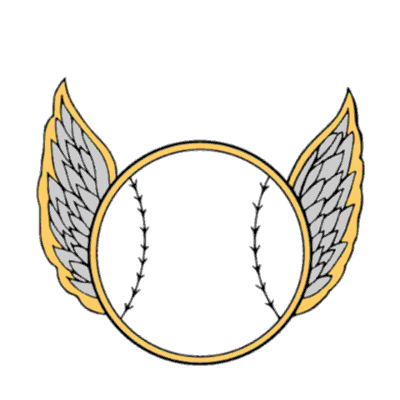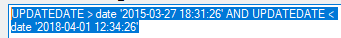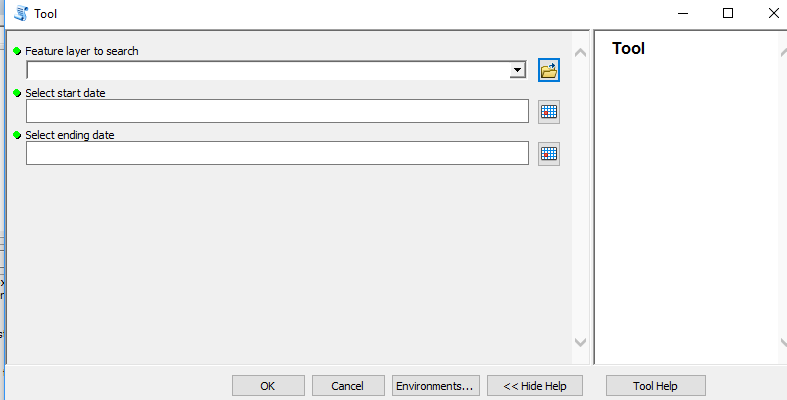# Use date timestamp field to select features

3001
10
04-29-2019 12:00 PMby Anonymous User
Not applicable

Hello:

I want a user to select a date range which will select all features in that range based on the date field. Any advice?

1 Solution

Accepted SolutionsbyMVP Regular Contributor

I was doing some experimenting with a Python toolbox and came up with the following code:

``````import arcpy
import datetime

class Toolbox(object):
def __init__(self):
"""Define the toolbox (the name of the toolbox is the name of the
.pyt file)."""
self.label = "Toolbox"
self.alias = ""

# List of tool classes associated with this toolbox
self.tools = [Tool]

class Tool(object):
def __init__(self):
"""Define the tool (tool name is the name of the class)."""
self.label = "Tool"
self.description = ""
self.canRunInBackground = False

def getParameterInfo(self):
"""Define parameter definitions"""

feature = arcpy.Parameter(
displayName = "Feature layer to search",
name = "feature",
datatype = "GPFeatureLayer",
parameterType = "Required",
direction = "Input")

startDate = arcpy.Parameter(
displayName = "Select start date",
name = "startDate",
datatype = "GPDate",
parameterType = "Required",
direction = "Input")

endDate = arcpy.Parameter(
displayName = "Select ending date",
name = "endDate",
datatype = "GPDate",
parameterType = "Required",
direction = "Input")

params = None
return [ feature, startDate, endDate ]

"""Set whether tool is licensed to execute."""
return True

def updateParameters(self, parameters):
"""Modify the values and properties of parameters before internal
validation is performed.  This method is called whenever a parameter
has been changed."""
return

def updateMessages(self, parameters):
"""Modify the messages created by internal validation for each tool
parameter.  This method is called after internal validation."""
return

def execute(self, parameters, messages):
"""The source code of the tool."""

# the addMessages are for debugging and can be removed

# one way to set the time portion
# startDate = '{} 00:00:00'.format(parameters.value.strftime("%Y-%m-%d"))
# endDate = '{} 23:59:59'.format(parameters.value.strftime("%Y-%m-%d"))

startDate = parameters.value.strftime("%Y-%m-%d %H:%M:%S")
endDate = parameters.value.strftime("%Y-%m-%d %H:%M:%S")

where = "DateTime BETWEEN DATE '{}' AND DATE '{}'".format(startDate, endDate)

arcpy.SelectLayerByAttribute_management(parameters.valueAsText, "NEW_SELECTION", where_clause=where)

return‍‍‍‍‍‍‍‍‍‍‍‍‍‍‍‍‍‍‍‍‍‍‍‍‍‍‍‍‍‍‍‍‍‍‍‍‍‍‍‍‍‍‍‍‍‍‍‍‍‍‍‍‍‍‍‍‍‍‍‍‍‍‍‍‍‍‍‍‍‍‍‍‍‍‍‍‍‍‍‍‍‍‍‍‍‍``````

There are lots of addMessage() for debugging.  At line 72, I used the type function to verify that the parameter being used was a datetime type.   In addition I came across this topic: arcpy script tool - format parameter Date data type. It may provide some additional insight in how date/time works in a Python script or toolbox.  Hope this helps.

10 RepliesbyMVP Esteemed Contributor

You saw this? because it depends on what you are querying as wellby Anonymous User
Not applicable

How can you use this in a python script?by Anonymous User
Not applicable

Is there a reference guide for that?byMVP Esteemed Contributor

I think your'e going to have to try out a few expressions to see how it works.  Looking at the reference Dan provides, there may be some fine tuning for your particular data base.  In a general sense:

where  YourDateField => someDate and YourDateField <= someOtherdate

The trick will be in how to format someDate and someOtherdate...

That should just about do it....byMVP Regular Contributor

Hi Brandon Price‌,

Looking for a Python example? You may want to have a look at this video, just to start somewhere:

Writing Your Own Python DateTime Functions in the Arcmap Field Calculator

HTH,

Egge-JanbyMVP Regular Contributor

'BETWEEN' will also work in date queries.  For example with a file geodatabase:

``````where = "DateTime BETWEEN DATE '2019-04-01 00:00:00' AND DATE '2019-04-30 23:59:59'"

with arcpy.da.SearchCursor(feature, ['DateTime'], where_clause=where) as cursor:
for row in cursor:
print row‍‍‍‍‍‍‍‍‍‍``````

You will need to check the SQL documentation for the type of geodatabase you are working with.   And the datetime module can also be very helpful.

``````from datetime import datetime, timedelta

date_past = datetime.now() - timedelta(days=180)

wc = "DateField < DATE '{}'".format(date_past.strftime("%Y-%m-%d %H:%M:%S"))

print wc‍‍‍‍‍‍‍‍‍‍‍‍‍‍‍‍‍‍‍‍‍``````by Anonymous User
Not applicable

I created a python toolbox and I am trying to recreate the select by attributes query below within it in the Start Date and end parameters.

Toolbox (user view):select by attributes query:This is the unfinished code:

import arcpy, os,sys
geoName = sys.argv
startDate = sys.argv
endDate = sys.argv

desiredDate =
query = startDate and e

I think maybe I need to convert the current date field (which is text) to a real date using the datetime module and then use the BETWEEN statement that Randy Burton suggested above to work for my class project.byMVP Regular Contributor

I was doing some experimenting with a Python toolbox and came up with the following code:

``````import arcpy
import datetime

class Toolbox(object):
def __init__(self):
"""Define the toolbox (the name of the toolbox is the name of the
.pyt file)."""
self.label = "Toolbox"
self.alias = ""

# List of tool classes associated with this toolbox
self.tools = [Tool]

class Tool(object):
def __init__(self):
"""Define the tool (tool name is the name of the class)."""
self.label = "Tool"
self.description = ""
self.canRunInBackground = False

def getParameterInfo(self):
"""Define parameter definitions"""

feature = arcpy.Parameter(
displayName = "Feature layer to search",
name = "feature",
datatype = "GPFeatureLayer",
parameterType = "Required",
direction = "Input")

startDate = arcpy.Parameter(
displayName = "Select start date",
name = "startDate",
datatype = "GPDate",
parameterType = "Required",
direction = "Input")

endDate = arcpy.Parameter(
displayName = "Select ending date",
name = "endDate",
datatype = "GPDate",
parameterType = "Required",
direction = "Input")

params = None
return [ feature, startDate, endDate ]

"""Set whether tool is licensed to execute."""
return True

def updateParameters(self, parameters):
"""Modify the values and properties of parameters before internal
validation is performed.  This method is called whenever a parameter
has been changed."""
return

def updateMessages(self, parameters):
"""Modify the messages created by internal validation for each tool
parameter.  This method is called after internal validation."""
return

def execute(self, parameters, messages):
"""The source code of the tool."""

# the addMessages are for debugging and can be removed

# one way to set the time portion
# startDate = '{} 00:00:00'.format(parameters.value.strftime("%Y-%m-%d"))
# endDate = '{} 23:59:59'.format(parameters.value.strftime("%Y-%m-%d"))

startDate = parameters.value.strftime("%Y-%m-%d %H:%M:%S")
endDate = parameters.value.strftime("%Y-%m-%d %H:%M:%S")

where = "DateTime BETWEEN DATE '{}' AND DATE '{}'".format(startDate, endDate)

arcpy.SelectLayerByAttribute_management(parameters.valueAsText, "NEW_SELECTION", where_clause=where)

return‍‍‍‍‍‍‍‍‍‍‍‍‍‍‍‍‍‍‍‍‍‍‍‍‍‍‍‍‍‍‍‍‍‍‍‍‍‍‍‍‍‍‍‍‍‍‍‍‍‍‍‍‍‍‍‍‍‍‍‍‍‍‍‍‍‍‍‍‍‍‍‍‍‍‍‍‍‍‍‍‍‍‍‍‍‍``````

There are lots of addMessage() for debugging.  At line 72, I used the type function to verify that the parameter being used was a datetime type.   In addition I came across this topic: arcpy script tool - format parameter Date data type. It may provide some additional insight in how date/time works in a Python script or toolbox.  Hope this helps.by Anonymous User
Not applicable

Hello Randy:

This is great. Thanks for your effort. This is what I got:I apologize. I am having trouble with the where clause and getting it to synchronize with my layer. The field that I am using  is called UPDATEDATE. I tried a variety of ways to add my field to the where clause and was unsuccessful. My coworkers version is similar to yours although he is using this query variable instead of the where clause which works:

query = '"UPDATEDATE" < date \'{0}\' and "UPDATEDATE" > date \'{1}\''.format(endDT,startDT)

This is his full version:

import arcpy,datetime
#from datetime import datetime as dt

FC = arcpy.GetParameterAsText(0)
FC_1 = FC.replace("\\","/")
statrDate = arcpy.GetParameterAsText(1)
endDate = arcpy.GetParameterAsText(2)

startDT = datetime.datetime.strptime(statrDate,'%m/%d/%Y %I:%M:%S %p')
endDT = datetime.datetime.strptime(endDate,'%m/%d/%Y %I:%M:%S %p')

outFC = arcpy.GetParameterAsText(3)
outFC_1 = outFC.replace("\\","/")
query = '"UPDATEDATE" < date \'{0}\' and "UPDATEDATE" > date \'{1}\''.format(endDT,startDT)
arcpy.Select_analysis(FC_1,outFC_1,query)

The date variables look similar to yours (startDT and endDT)

Brandon